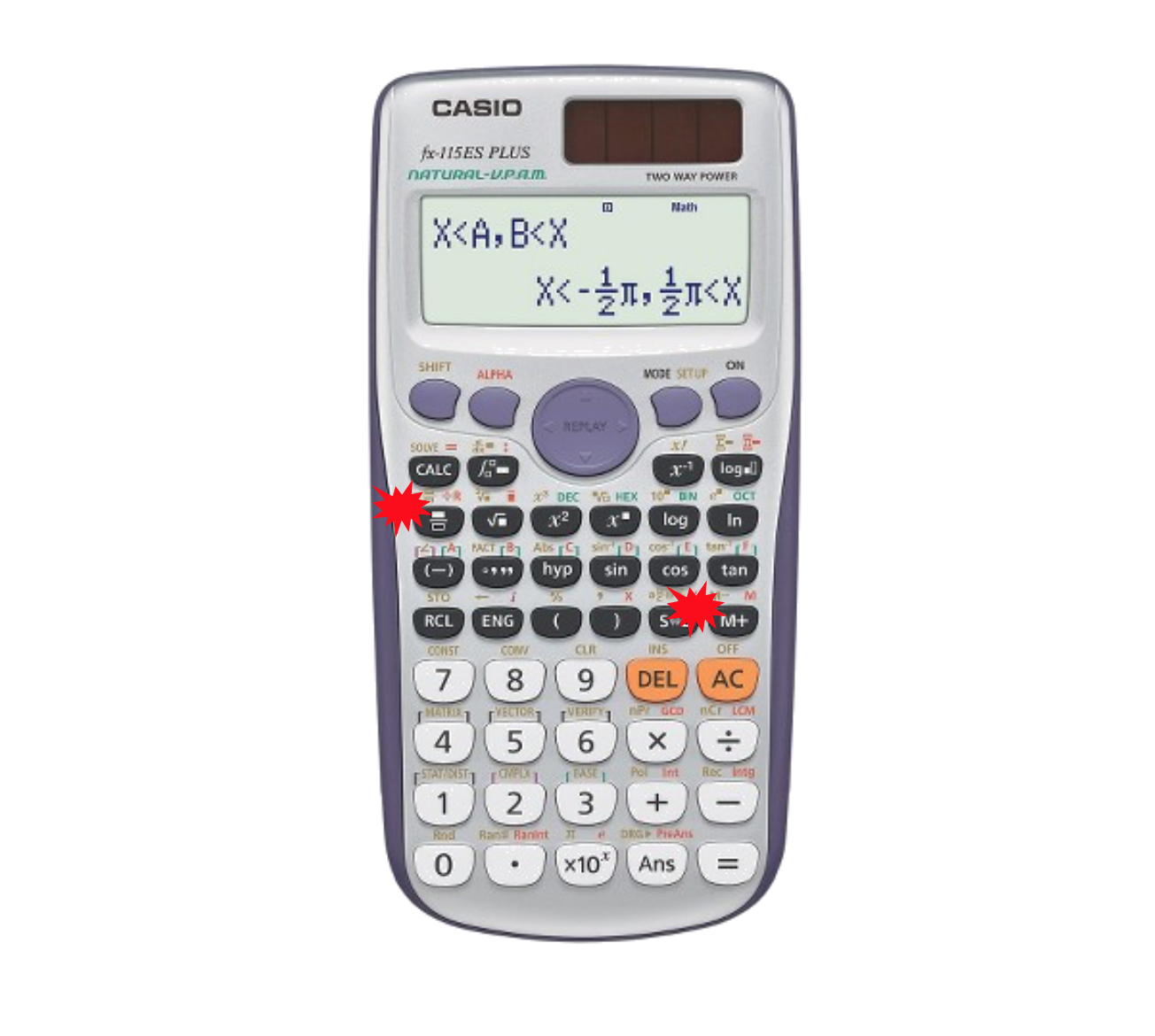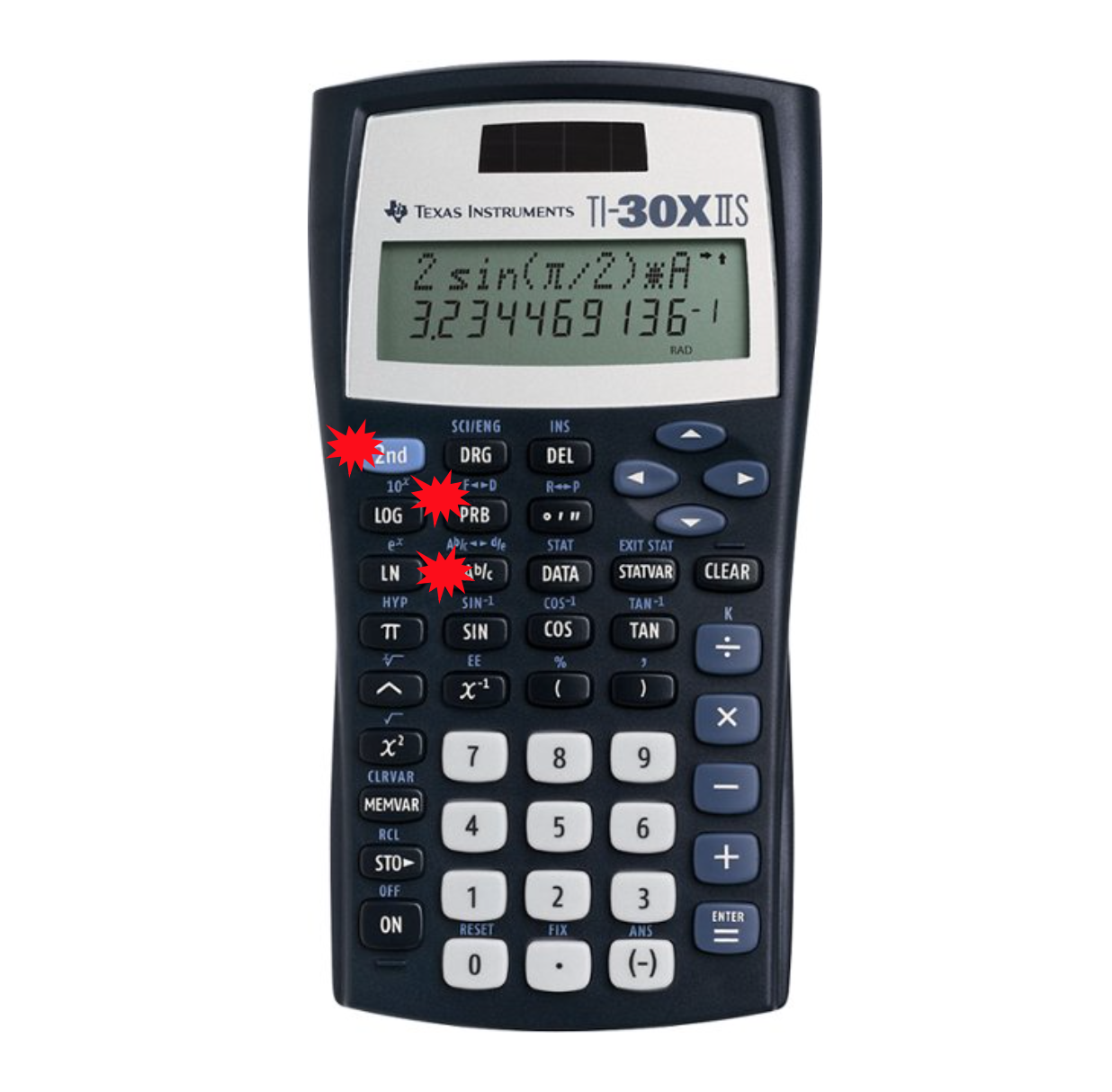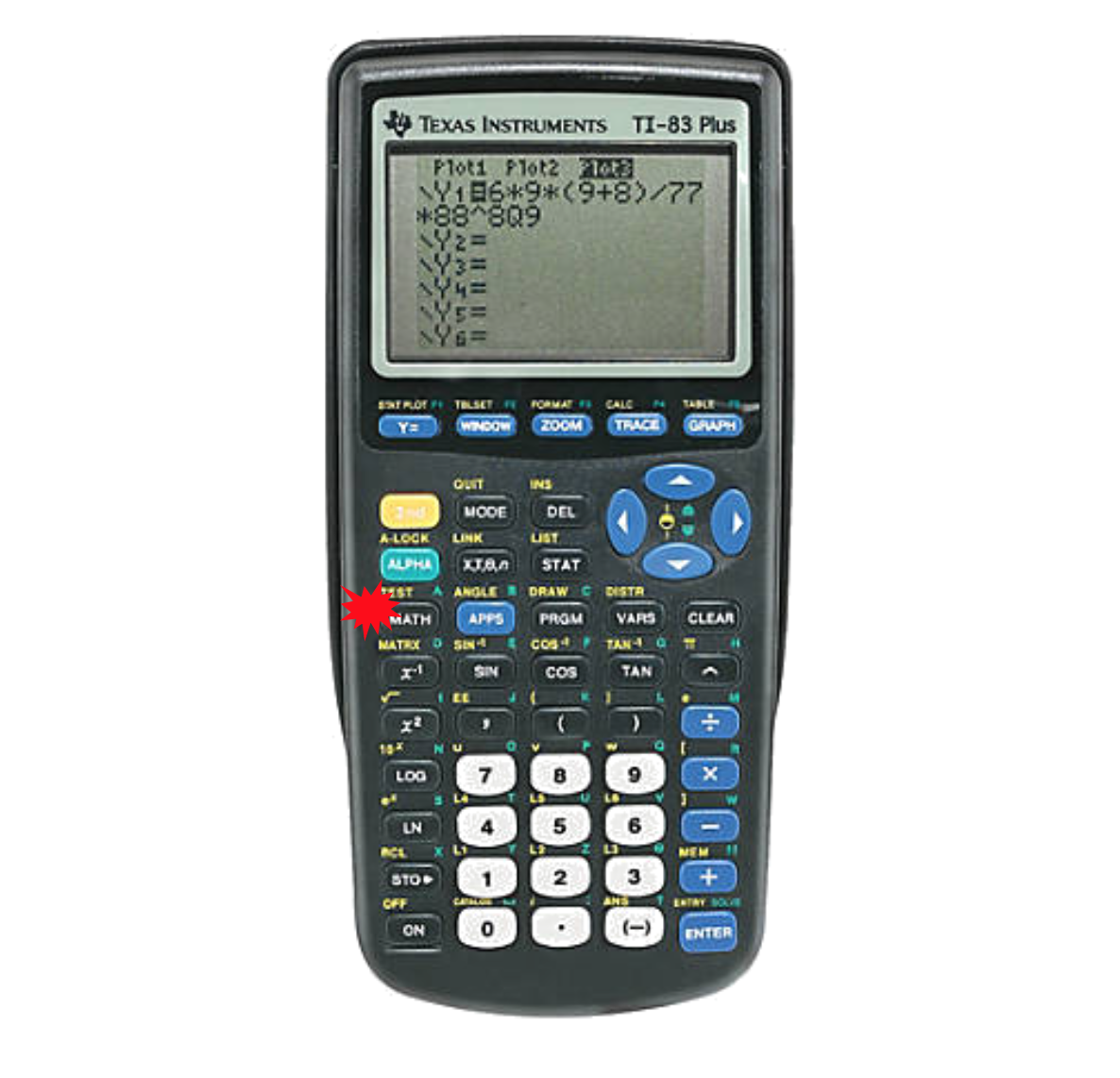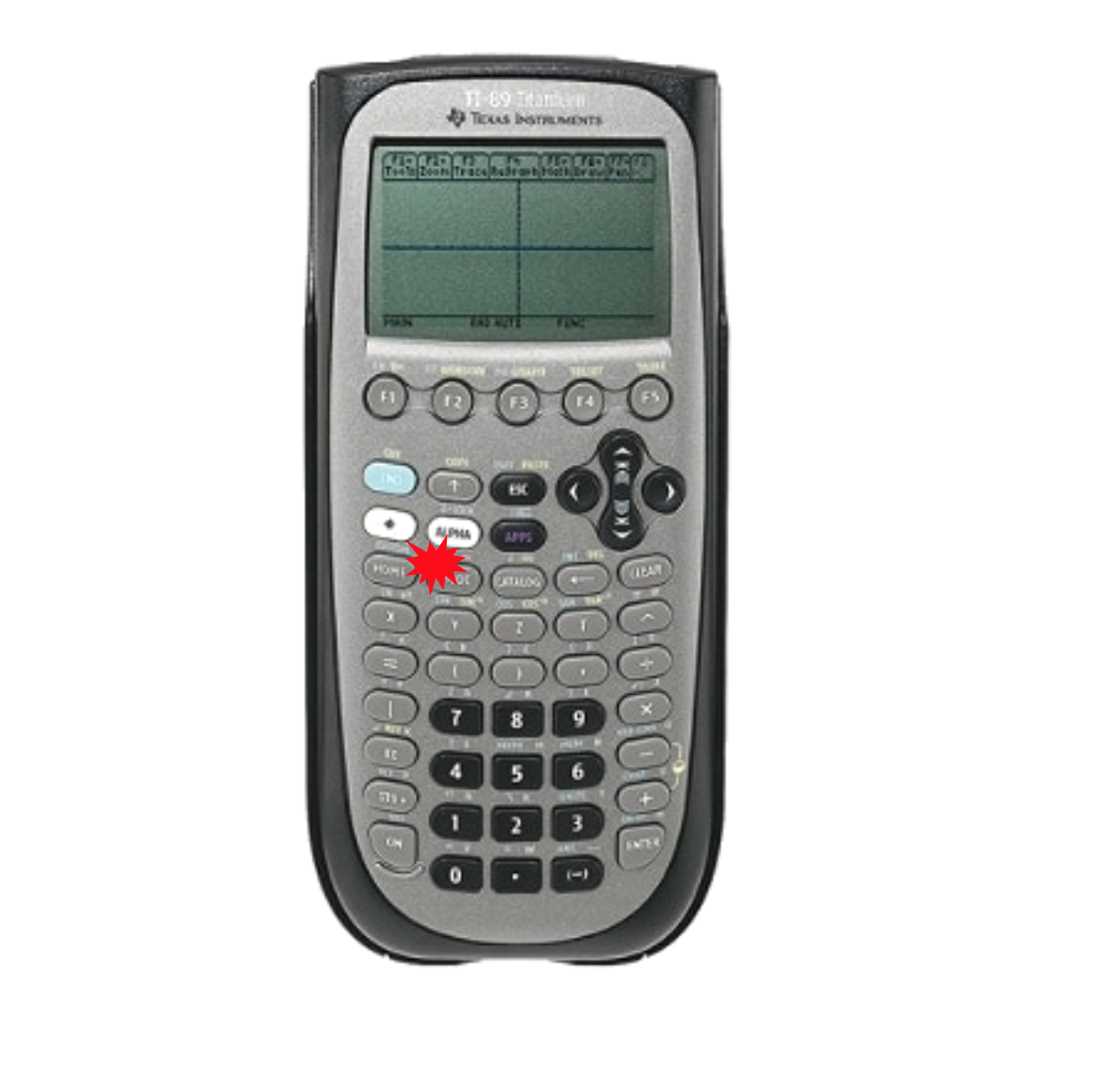# Online Math Tutoring: Fractions on Popular Student Calculators

Many students struggle with fractions and fraction manipulation.  In particular, if they have become reliant on a calculator for their operations it can be difficult to work with fractions.  By default, most calculators output decimals rather than fractions.  This leads to many teachers emphasizing working with fractions without a calculator.

While I agree that working through fractions without a calculator is more conducive to learning and understanding fractions, it does not mean that a calculator cannot be extremely effective in confirming your answers along the way.

First, it is important to recognize that many calculators that students own or have access to have built-in fraction functionality.  This means that the calculator can present your answers or problems in fractional form just like how they’d look if you wrote them out.

Here, we’ll show some popular calculators and how to work with fractions with them, including the various buttons you may need to press.Many Casio calculators do make working with fractions easy for you.  They output many answers already in a fractional form (you can change this in the settings under “mode” if you don’t want this process to be automatic) and have two main buttons to work with fractions.

The first button, on the left, lets you type in fractions just how you would write them.  The second button, on the right, lets you convert between fractions and decimals.  Note that pressings “shift” and then pressing the SóD button can also even give you access to mixed numbers.Next, we have a Texas Instruments scientific calculator.  This model does not make fractions quite as easy as most Casio models, but the same functions are still possible.  Note that the “2nd” button in the top-left gives access to every button’s secondary function.  Here, pressing “2nd" and then the top indicated button will convert your answers from fractions to decimal and vice-versa.  The button beneath it allows you to type fractions as well.  Using the “2nd" key for this button gives access to mixed numbers.Next, the most popular graphing calculators in schools tend to be the Texas Instruments TI-83 and 84 and similar variants.  While these calculators have more options and functions than the scientific calculators above, they do make fraction functionality more difficult to find.  For these graphing calculators, get used to hitting the buttons on the top half of the calculator to open various menus.  Here, we want to hit the “math” button.  The top option under this math menu will convert your decimals to fractions.  Many students learn this as hitting “math, enter, enter” with the calculator.Finally, we have a newer calculator and variations that are growing in popularity which are the TI-89 graphing calculator versions.  Unlike most graphing calculators, the 89 actually prefers to give you fractions over decimals.  To stop this automatic process, click the “mode” button and change the exact/approximate setting to approximate.  This will output decimals by default while exact will give you the fractions already that you may want

Understanding your calculator and being able to use it quickly and effectively is a valuable skill for math students.  While you should be careful not to become overly reliant on it, it can be a valuable tool to help you check answers and perform various computations quickly and accurately.  Whichever your calculator, gets used to using it learning the helpful tools it provides like fractional inputs and outputs.

Michael C. is currently an online private math, science, and standardized test tutor with TutorNerd in Irvine and Anaheim.